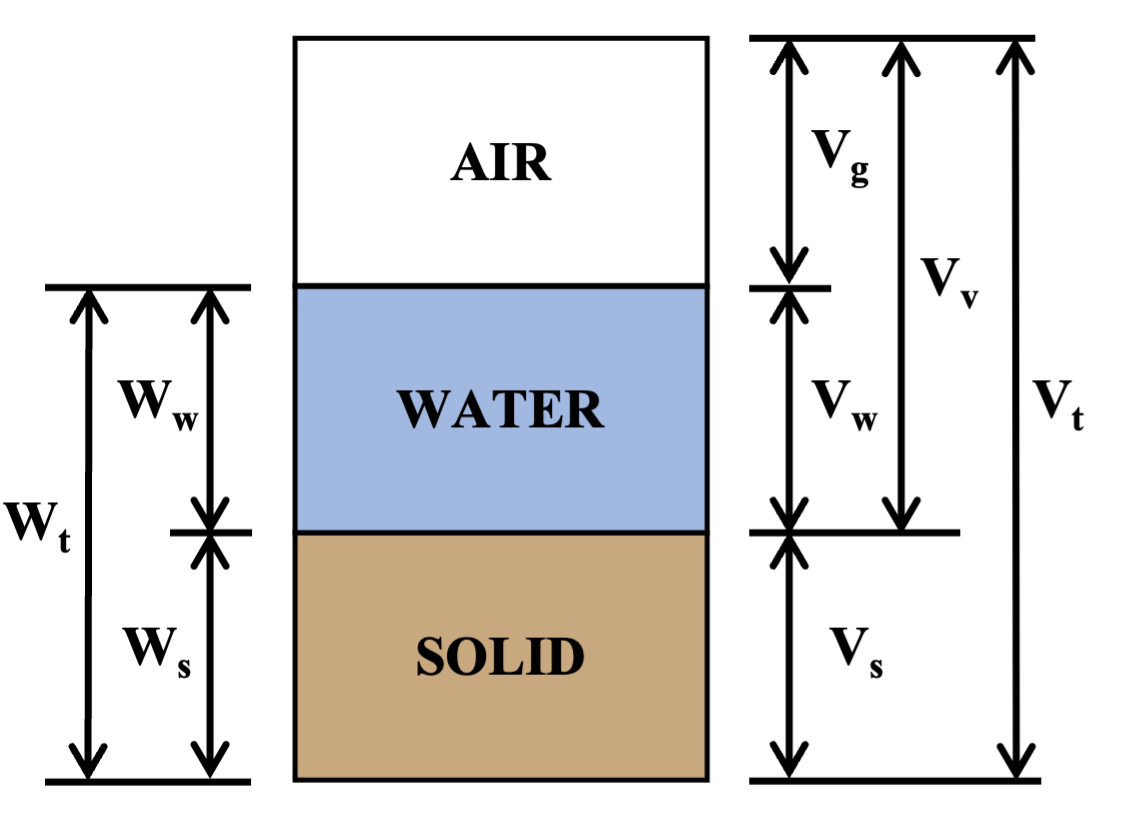# Total Unit Weight of Soil Based on Parameters

Materials: Soil PropertiesGiven the following parameters for a soil sample, determine the total unit weight:

Void Ratio $$e=0.66$$
Water Content $$w=20 \%$$
Weight of Solids in Sample: $$W_s=10 \ lb$$
Volume of Solids in Sample: $$\sout{V_s=260 \ in^3} \ \ V_s=100\ in^3$$
Unit Weight of Water: $$γ_w=62.4 \ pcf$$

A) 105 pcf

B) 115 pcf

C) 125 pcf

D) 140 pcf

Solution

$G_s= \frac{W_s}{V_s γ_w }= \frac{10 lb}{100 in^3×\frac{1}{(12 in)^3} ×62.4 pcf}=2.77$
$γ_t= \frac{(1+w) G_s γ_w}{1+e}$
$γ_t= \frac{(1+0.2)×2.77×62.4 pcf}{1+0.66}≈125 pcf$